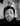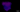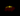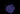Navigate back to the homepage

# Pulsating Volume in r3f and threeRick
November 18th, 2023 · 2 min readBelow is the code and example from codesandbox:

The premise is to spread planes in an area and make the planes lookat the camera (done automatically with points in Three). Then using a shaderMaterial modify the scale / gl_PointSize and colors of the planes uisng pre calculated pseudo normals.

Remember, when we use normals in light calculations it basically creates colours based on how the light interacts with the surface at this normal position. This means we get different colours based on the normals that we pre computed and passed to this shader.

Below is the main component for the shader.

```.css-1chxjt6{position:absolute;right:22px;top:24px;padding:8px 12px 7px;border-radius:5px;color:#6f7177;-webkit-transition:background 0.3s ease;transition:background 0.3s ease;}.css-1chxjt6:hover{background:rgba(255,255,255,0.07);}.css-1chxjt6[data-a11y="true"]:focus::after{content:"";position:absolute;left:-2%;top:-2%;width:104%;height:104%;border:2px solid var(--theme-ui-colors-accent,#6166DC);border-radius:5px;background:rgba(255,255,255,0.01);}@media (max-width:45.9375em){.css-1chxjt6{display:none;}}1const tex = useLoader(THREE.TextureLoader, "/test-3.3.png");2tex.encoding = THREE.LinearEncoding;3const orb = useLoader(THREE.TextureLoader, "/Smoke15Frames.png");4const pointsRef = useRef();5const shaderRef = useRef();6
7const shader = {8    uniforms: {9      positions: {10        value: tex,11      },12      orb: {13        value: orb,14      },15      time: {16        value: 0,17      },18    },19// shaders.....20};21
22let [positions, normals] = useMemo(() => {23  const positions = [];24  const normals = [];25
26  for (let i = 0; i < 400; i++) {27    const x = Math.random() * 30 - 15;28    const y = Math.random() * 30 - 15;29    const z = Math.random() * 30 - 15;30    positions.push(x, y, z);31
32    const normal = new THREE.Vector3(x, y, z).normalize();33    normals.push(normal.x, normal.y, normal.z);34  }35
36  return [new Float32Array(positions), new Float32Array(normals)];37}, []);38
39useFrame(({ clock }) => {40  if (shaderRef.current) {41    shaderRef.current.uniforms.time.value = clock.elapsedTime * 0.35;42  }43});44
45return (46  <group>47    <OrbitControls />48

So this is all pretty standard stuff.

We load some textures and apply to the planes to give the feel of transparency and fluffyness. This is done by loading the textures, and then passing them through a uniform to the shader and once in the shader we sample and output the alpha and mixed color.

Some key settings are as follows:

`1transparent={true}2alphaToCoverage3sizeAttenuation={false}4depthTest={true}5depthWrite={true}`

AlphaToCoverage is a technique described as:

`Enables alpha to coverage. Can only be used with MSAA-enabled contexts (meaning when the renderer was created with antialias parameter set to true). Default is false.`

This some what mitigates the transparency issue where bright colours shine through more darker ones.

We define a points object with custom attributes and then use a custom shaderMaterial, baring in mind that any attributes defined like this will have to use a typed array, as we have done in the useMemo function above

`1<points ref={pointsRef}>2  <bufferGeometry attach="geometry">3    <bufferAttribute4      attach="attributes-position"5      array={positions}6      count={positions.length / 3}7      itemSize={3}8    />9    <bufferAttribute10      attach="attributes-calNormal"11      array={normals}12      count={normals.length / 3.0}13      itemSize={3}14    />15  </bufferGeometry>16  <shaderMaterial17    attach="material"18    ref={shaderRef}19    vertexShader={shader.vertexShader}20    fragmentShader={shader.fragmentShader}21    uniforms={shader.uniforms}22    transparent={true}23    alphaToCoverage24    sizeAttenuation={false}25    depthTest={true}26    depthWrite={true}27  />28</points>`

We can then define attributes like:

`1attribute vec3 calNormal;`

The vertex shader is where we oscillate and scale up / down the planes over time, to give the pseudo effect of a moving volume / very fake basic dynamics. This is a neat way of mimicing volumes from a far. Doesnt necessarily look too good the closer up in 3D space you go.

```1uniform sampler2D positions;2uniform float time;3varying vec2 vUv;4varying vec3 vNormal;5varying float vDepth;6varying vec3 vPosition;7varying float vNoise;8attribute float index;9attribute vec3 calNormal;10
11\${noise}12
13void main () {14  15  float noise = Perlin3D(position * 0.041 + time);16
17  gl_PointSize = mix(160.0, 100.0 * 5.0, noise);18
19  vec4 worldPosition = modelMatrix * vec4(position, 1.0);20  vec4 cameraSpacePosition = viewMatrix * worldPosition;21  float distance = length(cameraSpacePosition.xyz);22  vDepth = distance;23  24  float adjustedPointSize = gl_PointSize * 120.0 / distance;  // Adjust this factor as needed25  gl_PointSize = adjustedPointSize;26
27  gl_Position = projectionMatrix * modelViewMatrix * vec4(position, 1.0) ;28  vNormal = calNormal;29  vDepth = gl_Position.z / gl_Position.w;30  vPosition = position;31  vNoise = noise;32}```

The point size or scale of the gl_PointSize is determined and modified such that it is quite big and it changes over time with a continuous noise function:

```1float noise = Perlin3D(position * 0.041 + time);2
3gl_PointSize = mix(160.0, 100.0 * 5.0, noise);```

(The time causes a new noise value every frame)

We slightly modify the gl_PointSize the closer it gets to the camera.

Then pass a fair few varying between shaders to be used in the fragment shader.

The fragment shader is where we utilize the normals and create colors for the planes:

```1uniform sampler2D orb;2uniform float time;3varying vec2 vUv;4varying vec3 vNormal;5varying float vDepth;6varying vec3 vPosition;7varying float vNoise;8
9
10\${noise}11
12void main () {13  14  float x = 256.0 / 1280.0;15  float y = 256.0 / 768.0;16
17  float total = 1280.0 / 256.0;18
19  float col = mod(1.0, total);20  float row = floor(1.0 / total);21
22  // Finally to UV texture coordinates23  vec2 uv = vec2(24    x * gl_PointCoord.x + col * x, 25    1.0 - y - row * y + y * gl_PointCoord.y26  );27
28  float alpha = texture2D(orb, uv).a;29
30  if (alpha < 0.01) discard;31
32  vec3 lightPosition = vec3(0.0,10.0,0.0);33
34  vec3 lightDir = normalize(lightPosition - vPosition.xyz);35
36  float distance = length(lightPosition - vPosition.xyz) * 2.030;37  float attenuation = 1.0 / (1.0 + 0.1 * distance + 0.01 * distance * distance);38
39  float intensity = dot(vNormal, lightDir);40
41  vec3 finalColor = vec3(intensity) * attenuation;42
43  // gl_FragColor = vec4( color.rgb, alpha);44  gl_FragColor = vec4(mix( finalColor + vec3(.20,0.,0.47),finalColor + vec3(.710,0.,0.47) , vNoise),alpha);45}```

The orb texture we sample from, we use some made up uvs:

```1float x = 256.0 / 1280.0;2float y = 256.0 / 768.0;3
4float total = 1280.0 / 256.0;5
6float col = mod(1.0, total);7float row = floor(1.0 / total);8
9// Finally to UV texture coordinates10vec2 uv = vec2(11  x * gl_PointCoord.x + col * x, 12  1.0 - y - row * y + y * gl_PointCoord.y13);14
15float alpha = texture2D(orb, uv).a;```

This is basically me playing around with it till I get something I like visually.

The light calculations is how a light source defined by the source position, interacts with this matertial:

```1vec3 lightPosition = vec3(0.0,10.0,0.0);2
3vec3 lightDir = normalize(lightPosition - vPosition.xyz);4
5float distance = length(lightPosition - vPosition.xyz) * 2.030;6float attenuation = 1.0 / (1.0 + 0.1 * distance + 0.01 * distance * distance);7
8float intensity = dot(vNormal, lightDir);9
10vec3 finalColor = vec3(intensity) * attenuation;```

Again this is me doing some basic light calculations all with pseudo values etc, just what looks good for me.

And then the final output color is:

`1gl_FragColor = vec4(mix( finalColor + vec3(.20,0.,0.47),finalColor + vec3(.710,0.,0.47) , vNoise),alpha);`

This is straight forward, we mix the final colour in both cases with an arbitary colour so we can get pulsating planes with differing colours, with a noise value for randomness and then using the alpha value we got earlier.

### More articles from theFrontDev### Order Indpendent transparency, webgl and opaqueness

In our latest blog post, we dissect the transparency issue within WebGl, shedding light on the technical intricacies that govern rendering. We explore the nuances of prioritizing opaque meshes, revealing how this hierarchy can impact the visual landscape. Discover how to navigate this digital terrain, ensuring that your web graphics and visualizations maintain fidelity in the face of transparency challenges, even when utilizing lights.

November 11th, 2023 · 7 min read### Abstract Blob Shader with Particles

Thefrontdev's take on an abstract blob which mimics an explosive type of event in space. Written in GLSL and using noise/particles/points with smoke textures on them. All built with the Three.js builtin materials and using onBeforeCompile to get all the standard light calculations from the builtin material.

August 8th, 2023 · 4 min read